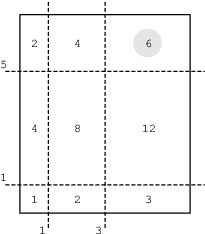Your browser (Unknown 0) is no longer supported. Some parts of the website may not work correctly. Please update your browser.

#### CuttingTheCake

Find the K-th piece of a cake in terms of size.

You are presented with a rectangular cake whose sides are of length X and Y. The cake has been cut into (N + 1)2 pieces by making N straight cuts along the first side and N straight cuts along the second side.

The cuts are represented by two non-empty arrays A and B consisting of N integers. More precisely, A[I] such that 0 ≤ I < N represents the position of a cut along the first side, and B[I] such that 0 ≤ I < N represents the position of a cut along the second side.

The goal is to find the K-th piece of cake in order of size, starting with the largest piece first. We will consider the size of a piece to be its area.

For example, a cake with sides X = 6, Y = 7 and arrays A and B such that:

A = 1 B = 1 A = 3 B = 5

is represented by the figure below.There are nine pieces of cake, and their consecutive sizes are: 12, 8, 6, 4, 4, 3, 2, 2, 1. In the figure above, the third piece of cake is highlighted; its size equals 6.

Write a function:

class Solution { public int solution(int X, int Y, int K, int[] A, int[] B); }

that, given three integers X, Y, K and two non-empty arrays A and B of N integers, returns the size of the K-th piece of cake.

For example, given:

X = 6 Y = 7 K = 3 A = 1 B = 1 A = 3 B = 5

the function should return 6, as explained above.

Write an efficient algorithm for the following assumptions:

• N is an integer within the range [1..40,000];
• X and Y are integers within the range [2..400,000,000];
• K is an integer within the range [1..(N+1)*(N+1)];
• each element of array A is an integer within the range [1..X-1];
• each element of array B is an integer within the range [1..Y-1];
• A[I − 1] < A[I] and B[I − 1] < B[I], for every I such that 0 < I < N;
• 1 ≤ A[I] − A[I − 1], B[I] − B[I − 1] ≤ 10,000, for every I such that 0 < I < N;
• 1 ≤ A, B, X − A[N − 1], Y − B[N − 1] ≤ 10,000.